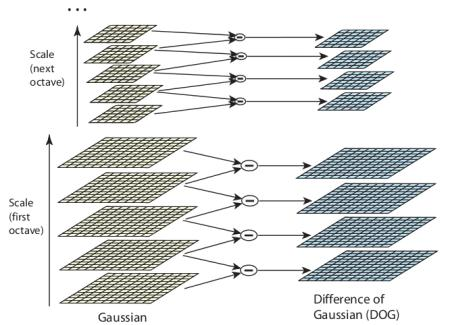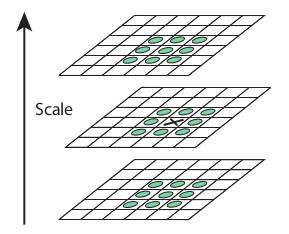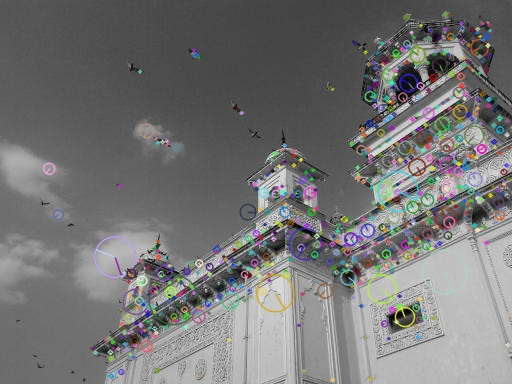# [SIFT]特征和描述符计算¶

SIFT(Scale Invariant Feature Transform，尺度不变特征变换)算子具有尺度和旋转不变性，同时对于仿射变换、噪声和光照具有很强的鲁棒性

## 原理¶

4步计算SIFT算子：

1. Scale-space Extrema Detection（尺度空间极值检测）
2. Keypoint Localization（关键点定位）
3. Orientation Assignment（方向赋值）
4. Keypoint Descriptor（关键点描述符）

### 尺度空间极值检测¶

1. 什么是尺度空间？

SIFT算子构建的尺度空间可以归纳为：对图像金字塔的每级图像执行不同大小的高斯核函数，以此来构建高斯金字塔模式的尺度空间

1. IoG和DoG

IoG指的是Laplacian of Gaussian，指的是图像和高斯核函数进行滤波后再进行拉普拉斯变换

Laplace算子计算公式如下：

∇^{2}f(x,y) = \frac {∂^{2}f}{∂x^{2}} + \frac {∂^{2}f}{∂y^{2}}

G_{σ}(x, y) = \frac {1}{\sqrt {2\pi σ^{2}}} \exp (- \frac {x^{2} + y^{2}}{2σ^{2}})

IoG计算公式如下：

Δ[G_{σ}(x,y)∗f(x,y)]=[ΔG_{σ}(x,y)]∗f(x,y)
LoG=ΔG_{σ}(x,y)=\frac {∂^{2}G_{σ}(x,y)}{∂x^{2}}+\frac {∂^{2}G_{σ}(x,y)}{∂y^{2}}=\frac {x^{2}+y^{2}−2σ^{2}}{σ^{4}}e^{−(x^{2}+y^{2})/2σ^{2}}

DoG指的是Difference of Gaussian，通过不同$σ$值的高斯滤波图像之间的差分来模拟IoG1. 如何检测极值### 关键点定位¶

• 设置对比度阈值（contrastThreshold，文中为0.3），用于去除小于该阈值的极值点
• 设置边阈值（edgeThreshold，文中为10），对极值点使用$2\times 2$ Hessian矩阵计算主曲率，如果大于边阈值，那么舍弃该极值点

## Python实现¶

• 创建SIFT对象
• 检测SIFT关键点
• 检测SIFT描述符
• 绘制SIFT关键点

### 创建SIFT对象¶

static Ptr<SIFT> cv::SIFT::create   (   int     nfeatures = 0,
int     nOctaveLayers = 3,
double      contrastThreshold = 0.04,
double      edgeThreshold = 10,
double      sigma = 1.6
)

• nfeatures：保留的关键点个数（默认所有）。其通过局部对比度进行排序
• nOctaveLayers：每一级的DoG个数，默认为3
• contrastThreshold：对比度阈值
• edgeThreshold：边阈值
• sigma：应用于第0级金字塔的高斯因子，如果使用a weak camera with soft lenses，可以减小该值

### 检测SIFT关键点并绘制¶

• 创建sift对象
• 调用detect函数检测关键点
• 调用drawKeypoints函数绘制关键点

detect函数计算后返回关键点列表，每个关键点类型为OpenCV自定义的KeyPoint

import numpy as np
import cv2 as cv

def sift_keypoint_draw():
img = cv.imread('home.jpg')
gray = cv.cvtColor(img, cv.COLOR_BGR2GRAY)

sift = cv.xfeatures2d.SIFT_create()
kp = sift.detect(gray, None)

cv.drawKeypoints(gray, kp, img, flags=cv.DRAW_MATCHES_FLAGS_DRAW_RICH_KEYPOINTS)
cv.imwrite('sift_keypoints.jpg', img)• gray：表示输入图像
• kp：表示关键点
• img：表示输出图像

def sift_keypoint_draw():
img = cv.imread('home.jpg')
gray = cv.cvtColor(img, cv.COLOR_BGR2GRAY)

sift = cv.xfeatures2d.SIFT_create()
kp = sift.detect(img, None)

cv.drawKeypoints(img, kp, img, flags=cv.DRAW_MATCHES_FLAGS_DRAW_RICH_KEYPOINTS)
cv.imwrite('sift_keypoints.jpg', img)### 计算描述符¶

def sift_descriptor_compute():
img = cv.imread('home.jpg')
gray = cv.cvtColor(img, cv.COLOR_BGR2GRAY)

sift = cv.xfeatures2d.SIFT_create()
kp = sift.detect(gray, None)

kp, des = sift.compute(gray, kp)


### 同时检测关键点并计算描述符¶

keypoints, descriptors  =   cv.Feature2D.detectAndCompute(  image, mask[, descriptors[, useProvidedKeypoints]]  )

• img：输入图像
• mask：掩码，计算指定区域SIFT特征

def sift_keypoint_descriptor():
img = cv.imread('home.jpg')
gray = cv.cvtColor(img, cv.COLOR_BGR2GRAY)

sift = cv.xfeatures2d.SIFT_create()
kp, des = sift.detectAndCompute(gray, None)